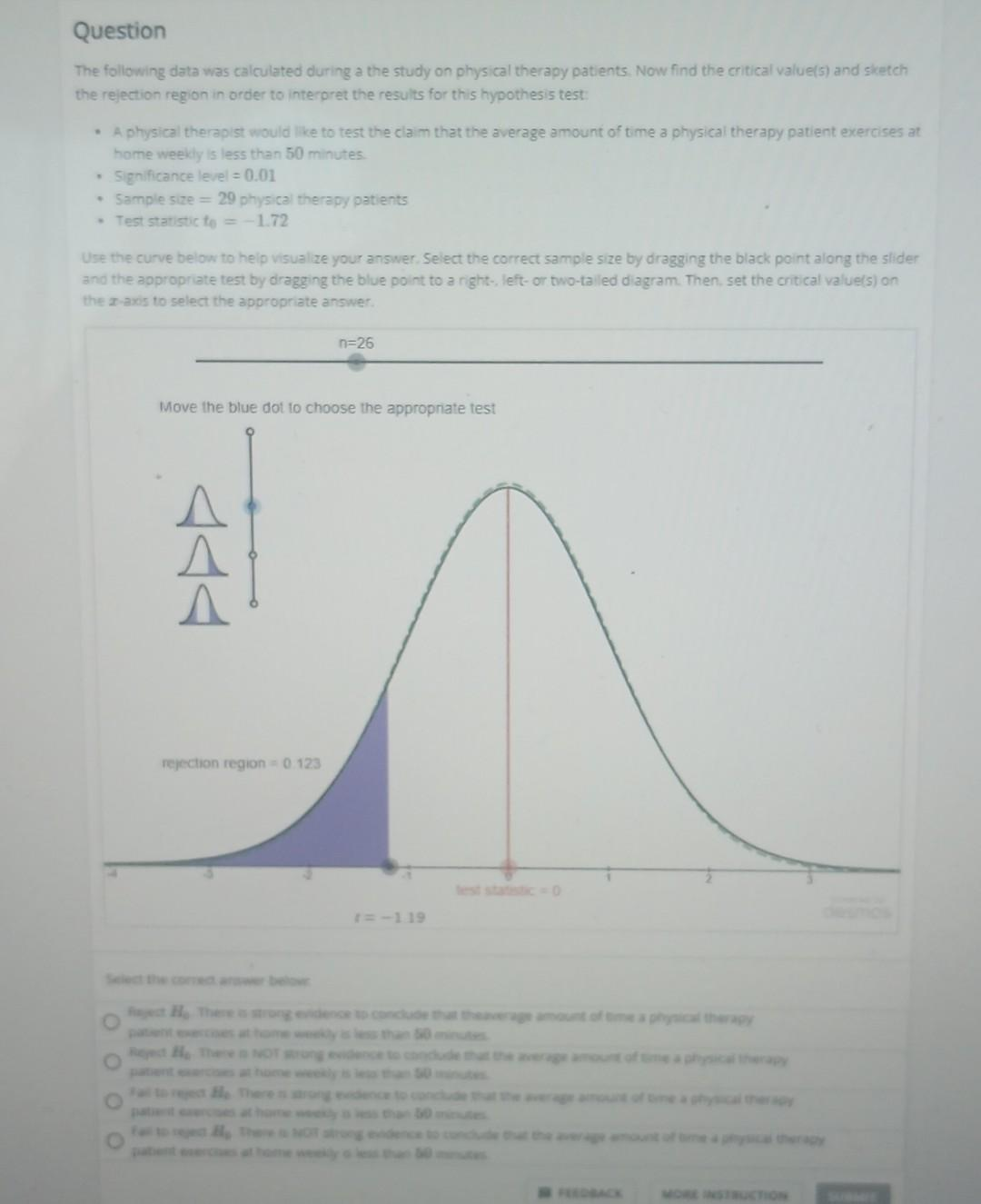Home / Expert Answers / Statistics and Probability / question-the-following-data-was-calculated-during-a-the-study-on-physical-therapy-patients-now-pa172

# (Solved): Question The following data was calculated during a the study on physical therapy patients. Now ...

Question
The following data was calculated during a the study on physical therapy patients. Now find the critical value(s) and sketch
the rejection region in order to interpret the results for this hypothesis test:
..
• A physical therapist would like to test the claim that the average amount of time a physical therapy patient exercises at
home weekly is less than 50 minutes.
.
• Significance level = 0.01
.
• Sample size 29 physical therapy patients
• Test statistic to = -1.72
Use the curve below to help visualize your answer. Select the correct sample size by dragging the black point along the slider
and the appropriate test by dragging the blue point to a right-, left- or two-tailed diagram. Then, set the critical value(s) on
the z-axis to select the appropriate answer.
O
Move the blue dot to choose the appropriate test
444
n=26
rejection region = 0.123
t=-1.19
test statistic = 0
O
Reject Ho. There is strong evidence to conclude that theaverage amount of time a physical therapy
patient exercises at home weekly is less than 50 minutes.
O
Reject Ho. There is NOT strong evidence to conclude that the average amount of time a physical therapy
patient exercises at home weekly is less than 50 minutes.
Fail to reject Ho. There is strong evidence to conclude that the average amount of time aphysical therapy
patient exercises at home weekly is less than 50 minutes.
Fail to reject He: There is NOT strong evidence to conclude that the average amount of time a physical therapy
patient exercises at home weekly is less than 50 minutes.
FEEDBACK
desmos
MORE INSTRUCTION

Question The following data was calculated during a the study on physical therapy patients. Now find the critical value(s) and sicetch the rejection region in order to interpret the results for this hypothesis test: - A physical therapist would like to test the claim that the average amount of time a physical therapy patient exercises at home weekly is less than 50 minutes. - Significance level - Sample size physical therapy patients - Test statistic Use the curve below to help visualize your answer. Select the correct sample size by dragging the black point aliong the slider and the appropriate test by dragging the blue point to a right-, left- or two-talled diagram. Then, set the critical value(s) on the -axis to select the appropriate answer. Move the blue dot to choose the appropriate test

We have an Answer from Expert